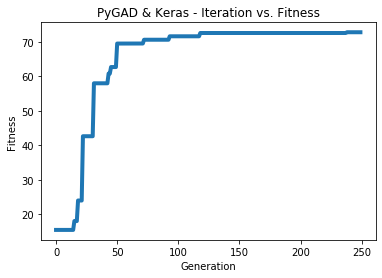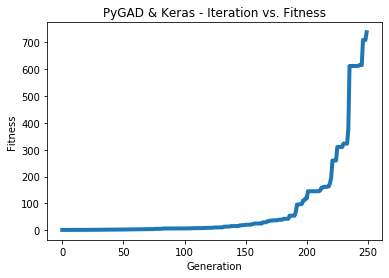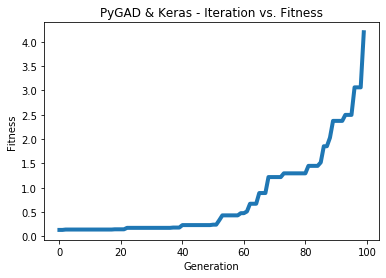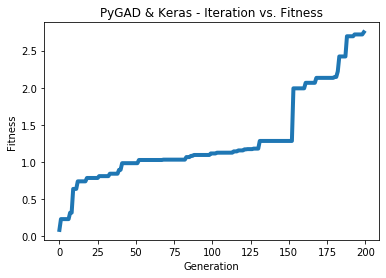# `pygad.kerasga` Module¶

The `pygad.kerarsga` module has helper a class and 2 functions to train Keras models using the genetic algorithm (PyGAD). The Keras model can be built either using the Sequential Model or the Functional API.

The contents of this module are:

1. `KerasGA`: A class for creating an initial population of all parameters in the Keras model.
2. `model_weights_as_vector()`: A function to reshape the Keras model weights to a single vector.
3. `model_weights_as_matrix()`: A function to restore the Keras model weights from a vector.
4. `predict()`: A function to make predictions based on the Keras model and a solution.

More details are given in the next sections.

# Steps Summary¶

The summary of the steps used to train a Keras model using PyGAD is as follows:

1. Create a Keras model.
2. Create an instance of the `pygad.kerasga.KerasGA` class.
3. Prepare the training data.
4. Build the fitness function.
5. Create an instance of the `pygad.GA` class.
6. Run the genetic algorithm.

# Create Keras Model¶

Before discussing training a Keras model using PyGAD, the first thing to do is to create the Keras model.

According to the Keras library documentation, there are 3 ways to build a Keras model:

PyGAD supports training the models created either using the Sequential Model or the Functional API.

Here is an example of a model created using the Sequential Model.

```import tensorflow.keras

input_layer  = tensorflow.keras.layers.Input(3)
dense_layer1 = tensorflow.keras.layers.Dense(5, activation="relu")
output_layer = tensorflow.keras.layers.Dense(1, activation="linear")

model = tensorflow.keras.Sequential()
```

This is the same model created using the Functional API.

```input_layer  = tensorflow.keras.layers.Input(3)
dense_layer1 = tensorflow.keras.layers.Dense(5, activation="relu")(input_layer)
output_layer = tensorflow.keras.layers.Dense(1, activation="linear")(dense_layer1)

model = tensorflow.keras.Model(inputs=input_layer, outputs=output_layer)
```

# `pygad.kerasga.KerasGA` Class¶

The `pygad.kerasga` module has a class named `KerasGA` for creating an initial population for the genetic algorithm based on a Keras model. The constructor, methods, and attributes within the class are discussed in this section.

## `__init__()`¶

The `pygad.kerasga.KerasGA` class constructor accepts the following parameters:

• `model`: An instance of the Keras model.
• `num_solutions`: Number of solutions in the population. Each solution has different parameters of the model.

## Instance Attributes¶

All parameters in the `pygad.kerasga.KerasGA` class constructor are used as instance attributes in addition to adding a new attribute called `population_weights`.

Here is a list of all instance attributes:

• `model`
• `num_solutions`
• `population_weights`: A nested list holding the weights of all solutions in the population.

## Methods in the `KerasGA` Class¶

This section discusses the methods available for instances of the `pygad.kerasga.KerasGA` class.

### `create_population()`¶

The `create_population()` method creates the initial population of the genetic algorithm as a list of solutions where each solution represents different model parameters. The list of networks is assigned to the `population_weights` attribute of the instance.

# Functions in the `pygad.kerasga` Module¶

This section discusses the functions in the `pygad.kerasga` module.

## `pygad.kerasga.model_weights_as_vector()`¶

The `model_weights_as_vector()` function accepts a single parameter named `model` representing the Keras model. It returns a vector holding all model weights. The reason for representing the model weights as a vector is that the genetic algorithm expects all parameters of any solution to be in a 1D vector form.

This function filters the layers based on the `trainable` attribute to see whether the layer weights are trained or not. For each layer, if its `trainable=False`, then its weights will not be evolved using the genetic algorithm. Otherwise, it will be represented in the chromosome and evolved.

The function accepts the following parameters:

• `model`: The Keras model.

It returns a 1D vector holding the model weights.

## `pygad.kerasga.model_weights_as_matrix()`¶

The `model_weights_as_matrix()` function accepts the following parameters:

1. `model`: The Keras model.
2. `weights_vector`: The model parameters as a vector.

It returns the restored model weights after reshaping the vector.

## `pygad.kerasga.predict()`¶

The `predict()` function makes a prediction based on a solution. It accepts the following parameters:

1. `model`: The Keras model.
2. `solution`: The solution evolved.
3. `data`: The test data inputs.

It returns the predictions for the data samples.

# Examples¶

This section gives the complete code of some examples that build and train a Keras model using PyGAD. Each subsection builds a different network.

## Example 1: Regression Example¶

The next code builds a simple Keras model for regression. The next subsections discuss each part in the code.

```import tensorflow.keras
import numpy

def fitness_func(solution, sol_idx):
global data_inputs, data_outputs, keras_ga, model

solution=solution,
data=data_inputs)

mae = tensorflow.keras.losses.MeanAbsoluteError()
abs_error = mae(data_outputs, predictions).numpy() + 0.00000001
solution_fitness = 1.0/abs_error

return solution_fitness

def callback_generation(ga_instance):
print("Generation = {generation}".format(generation=ga_instance.generations_completed))
print("Fitness    = {fitness}".format(fitness=ga_instance.best_solution()))

input_layer  = tensorflow.keras.layers.Input(3)
dense_layer1 = tensorflow.keras.layers.Dense(5, activation="relu")(input_layer)
output_layer = tensorflow.keras.layers.Dense(1, activation="linear")(dense_layer1)

model = tensorflow.keras.Model(inputs=input_layer, outputs=output_layer)

num_solutions=10)

# Data inputs
data_inputs = numpy.array([[0.02, 0.1, 0.15],
[0.7, 0.6, 0.8],
[1.5, 1.2, 1.7],
[3.2, 2.9, 3.1]])

# Data outputs
data_outputs = numpy.array([[0.1],
[0.6],
[1.3],
[2.5]])

num_generations = 250 # Number of generations.
num_parents_mating = 5 # Number of solutions to be selected as parents in the mating pool.
initial_population = keras_ga.population_weights # Initial population of network weights

num_parents_mating=num_parents_mating,
initial_population=initial_population,
fitness_func=fitness_func,
on_generation=callback_generation)

ga_instance.run()

# After the generations complete, some plots are showed that summarize how the outputs/fitness values evolve over generations.
ga_instance.plot_fitness(title="PyGAD & Keras - Iteration vs. Fitness", linewidth=4)

# Returning the details of the best solution.
solution, solution_fitness, solution_idx = ga_instance.best_solution()
print("Fitness value of the best solution = {solution_fitness}".format(solution_fitness=solution_fitness))
print("Index of the best solution : {solution_idx}".format(solution_idx=solution_idx))

# Make prediction based on the best solution.
solution=solution,
data=data_inputs)
print("Predictions : \n", predictions)

mae = tensorflow.keras.losses.MeanAbsoluteError()
abs_error = mae(data_outputs, predictions).numpy()
print("Absolute Error : ", abs_error)
```

### Create a Keras Model¶

According to the steps mentioned previously, the first step is to create a Keras model. Here is the code that builds the model using the Functional API.

```import tensorflow.keras

input_layer  = tensorflow.keras.layers.Input(3)
dense_layer1 = tensorflow.keras.layers.Dense(5, activation="relu")(input_layer)
output_layer = tensorflow.keras.layers.Dense(1, activation="linear")(dense_layer1)

model = tensorflow.keras.Model(inputs=input_layer, outputs=output_layer)
```

The model can also be build using the Keras Sequential Model API.

```input_layer  = tensorflow.keras.layers.Input(3)
dense_layer1 = tensorflow.keras.layers.Dense(5, activation="relu")
output_layer = tensorflow.keras.layers.Dense(1, activation="linear")

model = tensorflow.keras.Sequential()
```

### Create an Instance of the `pygad.kerasga.KerasGA` Class¶

The second step is to create an instance of the `pygad.kerasga.KerasGA` class. There are 10 solutions per population. Change this number according to your needs.

```import pygad.kerasga

num_solutions=10)
```

### Prepare the Training Data¶

The third step is to prepare the training data inputs and outputs. Here is an example where there are 4 samples. Each sample has 3 inputs and 1 output.

```import numpy

# Data inputs
data_inputs = numpy.array([[0.02, 0.1, 0.15],
[0.7, 0.6, 0.8],
[1.5, 1.2, 1.7],
[3.2, 2.9, 3.1]])

# Data outputs
data_outputs = numpy.array([[0.1],
[0.6],
[1.3],
[2.5]])
```

### Build the Fitness Function¶

The fourth step is to build the fitness function. This function must accept 2 parameters representing the solution and its index within the population.

The next fitness function returns the model predictions based on the current solution using the `predict()` function. Then, it calculates the mean absolute error (MAE) of the Keras model based on the parameters in the solution. The reciprocal of the MAE is used as the fitness value. Feel free to use any other loss function to calculate the fitness value.

```def fitness_func(solution, sol_idx):
global data_inputs, data_outputs, keras_ga, model

solution=solution,
data=data_inputs)

mae = tensorflow.keras.losses.MeanAbsoluteError()
abs_error = mae(data_outputs, predictions).numpy() + 0.00000001
solution_fitness = 1.0/abs_error

return solution_fitness
```

### Create an Instance of the `pygad.GA` Class¶

The fifth step is to instantiate the `pygad.GA` class. Note how the `initial_population` parameter is assigned to the initial weights of the Keras models.

```# Prepare the PyGAD parameters. Check the documentation for more information: https://pygad.readthedocs.io/en/latest/README_pygad_ReadTheDocs.html#pygad-ga-class
num_generations = 250 # Number of generations.
num_parents_mating = 5 # Number of solutions to be selected as parents in the mating pool.
initial_population = keras_ga.population_weights # Initial population of network weights

num_parents_mating=num_parents_mating,
initial_population=initial_population,
fitness_func=fitness_func,
on_generation=callback_generation)
```

### Run the Genetic Algorithm¶

The sixth and last step is to run the genetic algorithm by calling the `run()` method.

```ga_instance.run()
```

After the PyGAD completes its execution, then there is a figure that shows how the fitness value changes by generation. Call the `plot_fitness()` method to show the figure.

```ga_instance.plot_fitness(title="PyGAD & Keras - Iteration vs. Fitness", linewidth=4)
```

Here is the figure.To get information about the best solution found by PyGAD, use the `best_solution()` method.

```# Returning the details of the best solution.
solution, solution_fitness, solution_idx = ga_instance.best_solution()
print("Fitness value of the best solution = {solution_fitness}".format(solution_fitness=solution_fitness))
print("Index of the best solution : {solution_idx}".format(solution_idx=solution_idx))
```
```Fitness value of the best solution = 72.77768757825352
Index of the best solution : 0
```

The next code makes prediction using the `predict()` function to return the model predictions based on the best solution.

```# Fetch the parameters of the best solution.
solution=solution,
data=data_inputs)
print("Predictions : \n", predictions)
```
```Predictions :
[[0.09935353]
[0.63082725]
[1.2765523 ]
[2.4999595 ]]
```

The next code measures the trained model error.

```mae = tensorflow.keras.losses.MeanAbsoluteError()
abs_error = mae(data_outputs, predictions).numpy()
print("Absolute Error : ", abs_error)
```
```Absolute Error :  0.013740465
```

## Example 2: XOR Binary Classification¶

The next code creates a Keras model to build the XOR binary classification problem. Let’s highlight the changes compared to the previous example.

```import tensorflow.keras
import numpy

def fitness_func(solution, sol_idx):
global data_inputs, data_outputs, keras_ga, model

solution=solution,
data=data_inputs)

bce = tensorflow.keras.losses.BinaryCrossentropy()
solution_fitness = 1.0 / (bce(data_outputs, predictions).numpy() + 0.00000001)

return solution_fitness

def callback_generation(ga_instance):
print("Generation = {generation}".format(generation=ga_instance.generations_completed))
print("Fitness    = {fitness}".format(fitness=ga_instance.best_solution()))

# Build the keras model using the functional API.
input_layer  = tensorflow.keras.layers.Input(2)
dense_layer = tensorflow.keras.layers.Dense(4, activation="relu")(input_layer)
output_layer = tensorflow.keras.layers.Dense(2, activation="softmax")(dense_layer)

model = tensorflow.keras.Model(inputs=input_layer, outputs=output_layer)

# Create an instance of the pygad.kerasga.KerasGA class to build the initial population.
num_solutions=10)

# XOR problem inputs
data_inputs = numpy.array([[0, 0],
[0, 1],
[1, 0],
[1, 1]])

# XOR problem outputs
data_outputs = numpy.array([[1, 0],
[0, 1],
[0, 1],
[1, 0]])

num_generations = 250 # Number of generations.
num_parents_mating = 5 # Number of solutions to be selected as parents in the mating pool.
initial_population = keras_ga.population_weights # Initial population of network weights.

# Create an instance of the pygad.GA class
num_parents_mating=num_parents_mating,
initial_population=initial_population,
fitness_func=fitness_func,
on_generation=callback_generation)

# Start the genetic algorithm evolution.
ga_instance.run()

# After the generations complete, some plots are showed that summarize how the outputs/fitness values evolve over generations.
ga_instance.plot_fitness(title="PyGAD & Keras - Iteration vs. Fitness", linewidth=4)

# Returning the details of the best solution.
solution, solution_fitness, solution_idx = ga_instance.best_solution()
print("Fitness value of the best solution = {solution_fitness}".format(solution_fitness=solution_fitness))
print("Index of the best solution : {solution_idx}".format(solution_idx=solution_idx))

# Make predictions based on the best solution.
solution=solution,
data=data_inputs)
print("Predictions : \n", predictions)

# Calculate the binary crossentropy for the trained model.
bce = tensorflow.keras.losses.BinaryCrossentropy()
print("Binary Crossentropy : ", bce(data_outputs, predictions).numpy())

# Calculate the classification accuracy for the trained model.
ba = tensorflow.keras.metrics.BinaryAccuracy()
ba.update_state(data_outputs, predictions)
accuracy = ba.result().numpy()
print("Accuracy : ", accuracy)
```

Compared to the previous regression example, here are the changes:

• The Keras model is changed according to the nature of the problem. Now, it has 2 inputs and 2 outputs with an in-between hidden layer of 4 neurons.
```# Build the keras model using the functional API.
input_layer  = tensorflow.keras.layers.Input(2)
dense_layer = tensorflow.keras.layers.Dense(4, activation="relu")(input_layer)
output_layer = tensorflow.keras.layers.Dense(2, activation="softmax")(dense_layer)

model = tensorflow.keras.Model(inputs=input_layer, outputs=output_layer)
```
• The train data is changed. Note that the output of each sample is a 1D vector of 2 values, 1 for each class.
```# XOR problem inputs
data_inputs = numpy.array([[0, 0],
[0, 1],
[1, 0],
[1, 1]])

# XOR problem outputs
data_outputs = numpy.array([[1, 0],
[0, 1],
[0, 1],
[1, 0]])
```
• The fitness value is calculated based on the binary cross entropy.
```bce = tensorflow.keras.losses.BinaryCrossentropy()
solution_fitness = 1.0 / (bce(data_outputs, predictions).numpy() + 0.00000001)
```

After the previous code completes, the next figure shows how the fitness value change by generation.Here is some information about the trained model. Its fitness value is `739.24`, loss is `0.0013527311` and accuracy is 100%.

```Fitness value of the best solution = 739.2397344644013
Index of the best solution : 7

Predictions :
[[9.9694413e-01 3.0558957e-03]
[5.0176249e-04 9.9949825e-01]
[1.8470541e-03 9.9815291e-01]
[9.9999976e-01 2.0538971e-07]]

Binary Crossentropy :  0.0013527311

Accuracy :  1.0
```

## Example 3: Image Multi-Class Classification (Dense Layers)¶

Here is the code.

```import tensorflow.keras
import numpy

def fitness_func(solution, sol_idx):
global data_inputs, data_outputs, keras_ga, model

solution=solution,
data=data_inputs)

cce = tensorflow.keras.losses.CategoricalCrossentropy()
solution_fitness = 1.0 / (cce(data_outputs, predictions).numpy() + 0.00000001)

return solution_fitness

def callback_generation(ga_instance):
print("Generation = {generation}".format(generation=ga_instance.generations_completed))
print("Fitness    = {fitness}".format(fitness=ga_instance.best_solution()))

# Build the keras model using the functional API.
input_layer  = tensorflow.keras.layers.Input(360)
dense_layer = tensorflow.keras.layers.Dense(50, activation="relu")(input_layer)
output_layer = tensorflow.keras.layers.Dense(4, activation="softmax")(dense_layer)

model = tensorflow.keras.Model(inputs=input_layer, outputs=output_layer)

# Create an instance of the pygad.kerasga.KerasGA class to build the initial population.
num_solutions=10)

# Data inputs

# Data outputs
data_outputs = tensorflow.keras.utils.to_categorical(data_outputs)

num_generations = 100 # Number of generations.
num_parents_mating = 5 # Number of solutions to be selected as parents in the mating pool.
initial_population = keras_ga.population_weights # Initial population of network weights.

# Create an instance of the pygad.GA class
num_parents_mating=num_parents_mating,
initial_population=initial_population,
fitness_func=fitness_func,
on_generation=callback_generation)

# Start the genetic algorithm evolution.
ga_instance.run()

# After the generations complete, some plots are showed that summarize how the outputs/fitness values evolve over generations.
ga_instance.plot_fitness(title="PyGAD & Keras - Iteration vs. Fitness", linewidth=4)

# Returning the details of the best solution.
solution, solution_fitness, solution_idx = ga_instance.best_solution()
print("Fitness value of the best solution = {solution_fitness}".format(solution_fitness=solution_fitness))
print("Index of the best solution : {solution_idx}".format(solution_idx=solution_idx))

# Make predictions based on the best solution.
solution=solution,
data=data_inputs)
# print("Predictions : \n", predictions)

# Calculate the categorical crossentropy for the trained model.
cce = tensorflow.keras.losses.CategoricalCrossentropy()
print("Categorical Crossentropy : ", cce(data_outputs, predictions).numpy())

# Calculate the classification accuracy for the trained model.
ca = tensorflow.keras.metrics.CategoricalAccuracy()
ca.update_state(data_outputs, predictions)
accuracy = ca.result().numpy()
print("Accuracy : ", accuracy)
```

Compared to the previous binary classification example, this example has multiple classes (4) and thus the loss is measured using categorical cross entropy.

```cce = tensorflow.keras.losses.CategoricalCrossentropy()
solution_fitness = 1.0 / (cce(data_outputs, predictions).numpy() + 0.00000001)
```

### Prepare the Training Data¶

Before building and training neural networks, the training data (input and output) needs to be prepared. The inputs and the outputs of the training data are NumPy arrays.

The data used in this example is available as 2 files:

The data consists of 4 classes of images. The image shape is `(100, 100, 3)`. The number of training samples is 1962. The feature vector extracted from each image has a length 360.

Simply download these 2 files and read them according to the next code. Note that the class labels are one-hot encoded using the `tensorflow.keras.utils.to_categorical()` function.

```import numpy

data_outputs = tensorflow.keras.utils.to_categorical(data_outputs)
```

The next figure shows how the fitness value changes.Here are some statistics about the trained model.

```Fitness value of the best solution = 4.197464252185969
Index of the best solution : 0
Categorical Crossentropy :  0.23823906
Accuracy :  0.9852192
```

## Example 4: Image Multi-Class Classification (Conv Layers)¶

Compared to the previous example that uses only dense layers, this example uses convolutional layers to classify the same dataset.

Here is the complete code.

```import tensorflow.keras
import numpy

def fitness_func(solution, sol_idx):
global data_inputs, data_outputs, keras_ga, model

solution=solution,
data=data_inputs)

cce = tensorflow.keras.losses.CategoricalCrossentropy()
solution_fitness = 1.0 / (cce(data_outputs, predictions).numpy() + 0.00000001)

return solution_fitness

def callback_generation(ga_instance):
print("Generation = {generation}".format(generation=ga_instance.generations_completed))
print("Fitness    = {fitness}".format(fitness=ga_instance.best_solution()))

# Build the keras model using the functional API.
input_layer = tensorflow.keras.layers.Input(shape=(100, 100, 3))
conv_layer1 = tensorflow.keras.layers.Conv2D(filters=5,
kernel_size=7,
activation="relu")(input_layer)
max_pool1 = tensorflow.keras.layers.MaxPooling2D(pool_size=(5,5),
strides=5)(conv_layer1)
conv_layer2 = tensorflow.keras.layers.Conv2D(filters=3,
kernel_size=3,
activation="relu")(max_pool1)
flatten_layer  = tensorflow.keras.layers.Flatten()(conv_layer2)
dense_layer = tensorflow.keras.layers.Dense(15, activation="relu")(flatten_layer)
output_layer = tensorflow.keras.layers.Dense(4, activation="softmax")(dense_layer)

model = tensorflow.keras.Model(inputs=input_layer, outputs=output_layer)

# Create an instance of the pygad.kerasga.KerasGA class to build the initial population.
num_solutions=10)

# Data inputs

# Data outputs
data_outputs = tensorflow.keras.utils.to_categorical(data_outputs)

num_generations = 200 # Number of generations.
num_parents_mating = 5 # Number of solutions to be selected as parents in the mating pool.
initial_population = keras_ga.population_weights # Initial population of network weights.

# Create an instance of the pygad.GA class
num_parents_mating=num_parents_mating,
initial_population=initial_population,
fitness_func=fitness_func,
on_generation=callback_generation)

# Start the genetic algorithm evolution.
ga_instance.run()

# After the generations complete, some plots are showed that summarize how the outputs/fitness values evolve over generations.
ga_instance.plot_fitness(title="PyGAD & Keras - Iteration vs. Fitness", linewidth=4)

# Returning the details of the best solution.
solution, solution_fitness, solution_idx = ga_instance.best_solution()
print("Fitness value of the best solution = {solution_fitness}".format(solution_fitness=solution_fitness))
print("Index of the best solution : {solution_idx}".format(solution_idx=solution_idx))

# Make predictions based on the best solution.
solution=solution,
data=data_inputs)
# print("Predictions : \n", predictions)

# Calculate the categorical crossentropy for the trained model.
cce = tensorflow.keras.losses.CategoricalCrossentropy()
print("Categorical Crossentropy : ", cce(data_outputs, predictions).numpy())

# Calculate the classification accuracy for the trained model.
ca = tensorflow.keras.metrics.CategoricalAccuracy()
ca.update_state(data_outputs, predictions)
accuracy = ca.result().numpy()
print("Accuracy : ", accuracy)
```

Compared to the previous example, the only change is that the architecture uses convolutional and max-pooling layers. The shape of each input sample is 100x100x3.

```# Build the keras model using the functional API.
input_layer = tensorflow.keras.layers.Input(shape=(100, 100, 3))
conv_layer1 = tensorflow.keras.layers.Conv2D(filters=5,
kernel_size=7,
activation="relu")(input_layer)
max_pool1 = tensorflow.keras.layers.MaxPooling2D(pool_size=(5,5),
strides=5)(conv_layer1)
conv_layer2 = tensorflow.keras.layers.Conv2D(filters=3,
kernel_size=3,
activation="relu")(max_pool1)
flatten_layer  = tensorflow.keras.layers.Flatten()(conv_layer2)
dense_layer = tensorflow.keras.layers.Dense(15, activation="relu")(flatten_layer)
output_layer = tensorflow.keras.layers.Dense(4, activation="softmax")(dense_layer)

model = tensorflow.keras.Model(inputs=input_layer, outputs=output_layer)
```

### Prepare the Training Data¶

The data used in this example is available as 2 files:

The data consists of 4 classes of images. The image shape is `(100, 100, 3)` and there are 20 images per class for a total of 80 training samples. For more information about the dataset, check the Reading the Data section of the `pygad.cnn` module.

Simply download these 2 files and read them according to the next code. Note that the class labels are one-hot encoded using the `tensorflow.keras.utils.to_categorical()` function.

```import numpy

data_outputs = tensorflow.keras.utils.to_categorical(data_outputs)
```

The next figure shows how the fitness value changes.Here are some statistics about the trained model. The model accuracy is 75% after the 200 generations. Note that just running the code again may give different results.

```Fitness value of the best solution = 2.7462310258668805
Index of the best solution : 0
Categorical Crossentropy :  0.3641354
Accuracy :  0.75
```

To improve the model performance, you can do the following: